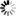Welcome to Central Library, SUST
Normal view

# Mathematics in Computing [electronic resource] : An Accessible Guide to Historical, Foundational and Application Contexts / by Gerard O’Regan.

Material type:TextPublisher: London : Springer London : Imprint: Springer, 2013Description: XX, 288 p. online resourceContent type:
• text
Media type:
• computer
Carrier type:
• online resource
ISBN:
• 9781447145349
Additional physical formats: Printed edition:: No titleDDC classification:
• 004.0151 23
LOC classification:
• QA76.9.M35
Online resources:
Contents:
Mathematics in Civilization -- Sets, Relations and Functions -- Logic -- Software Engineering -- Formal Methods -- Z Formal Specification Language -- Number Theory -- Cryptography -- Coding Theory -- Language Theory and Semantics -- Computability and Decidability -- Probability, Statistics and Software Reliability -- Matrix Theory -- Complex Numbers and Quaternions -- Calculus -- Graph Theory.
Summary: From the earliest examples of computation to the digital devices that are ubiquitous in modern society, the application of mathematics to computing has underpinned the technology that has built our world. This clearly written and enlightening textbook/reference provides a concise, introductory guide to the key mathematical concepts and techniques used by computer scientists. Spanning a wide range of topics – from number theory to software engineering – the book demonstrates the practical computing applications behind seemingly abstract ideas. The work of important figures such as Alan Turing and Robert Floyd are also discussed, highlighting how the theory has been informed by historical developments. Topics and features: Ideal for self-study, offering many pedagogical features such as chapter-opening key topics, chapter introductions and summaries, review questions, and a glossary Places our current state of knowledge within the context of the contributions made by early civilizations, such as the ancient Babylonians, Egyptians and Greeks Examines the building blocks of mathematics, including sets, relations and functions Presents an introduction to logic, formal methods and software engineering Explains the fundamentals of number theory, and its application in cryptography Describes the basics of coding theory, language theory, and graph theory Discusses the concept of computability and decideability Includes concise coverage of calculus, probability and statistics, matrices, complex numbers and quaternions This engaging and easy-to-understand book will appeal to students of computer science wishing for an overview of the mathematics used in computing, and to mathematicians curious about how their subject is applied in the field of computer science. The book will also capture the interest of the motivated general reader.
Tags from this library: No tags from this library for this title.
Star ratingsAverage rating: 0.0 (0 votes)
No physical items for this record

Mathematics in Civilization -- Sets, Relations and Functions -- Logic -- Software Engineering -- Formal Methods -- Z Formal Specification Language -- Number Theory -- Cryptography -- Coding Theory -- Language Theory and Semantics -- Computability and Decidability -- Probability, Statistics and Software Reliability -- Matrix Theory -- Complex Numbers and Quaternions -- Calculus -- Graph Theory.

From the earliest examples of computation to the digital devices that are ubiquitous in modern society, the application of mathematics to computing has underpinned the technology that has built our world. This clearly written and enlightening textbook/reference provides a concise, introductory guide to the key mathematical concepts and techniques used by computer scientists. Spanning a wide range of topics – from number theory to software engineering – the book demonstrates the practical computing applications behind seemingly abstract ideas. The work of important figures such as Alan Turing and Robert Floyd are also discussed, highlighting how the theory has been informed by historical developments. Topics and features: Ideal for self-study, offering many pedagogical features such as chapter-opening key topics, chapter introductions and summaries, review questions, and a glossary Places our current state of knowledge within the context of the contributions made by early civilizations, such as the ancient Babylonians, Egyptians and Greeks Examines the building blocks of mathematics, including sets, relations and functions Presents an introduction to logic, formal methods and software engineering Explains the fundamentals of number theory, and its application in cryptography Describes the basics of coding theory, language theory, and graph theory Discusses the concept of computability and decideability Includes concise coverage of calculus, probability and statistics, matrices, complex numbers and quaternions This engaging and easy-to-understand book will appeal to students of computer science wishing for an overview of the mathematics used in computing, and to mathematicians curious about how their subject is applied in the field of computer science. The book will also capture the interest of the motivated general reader.

There are no comments on this title.